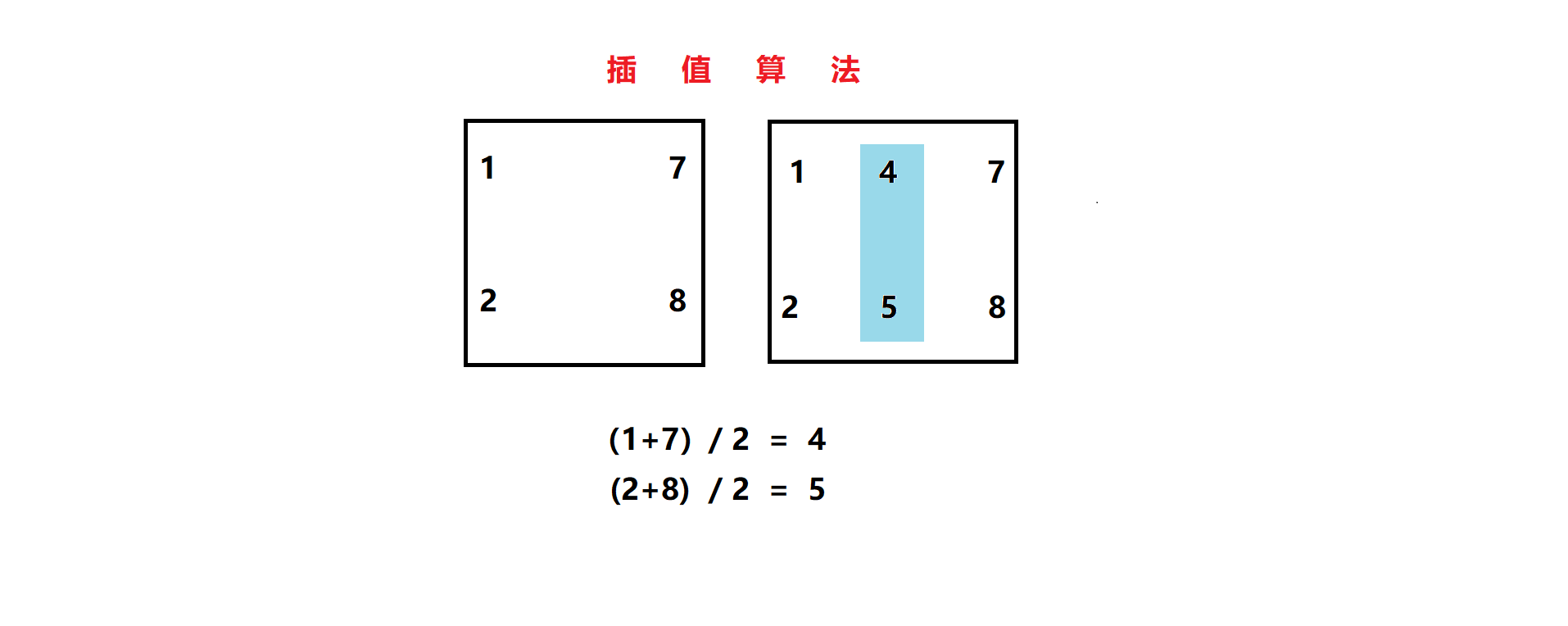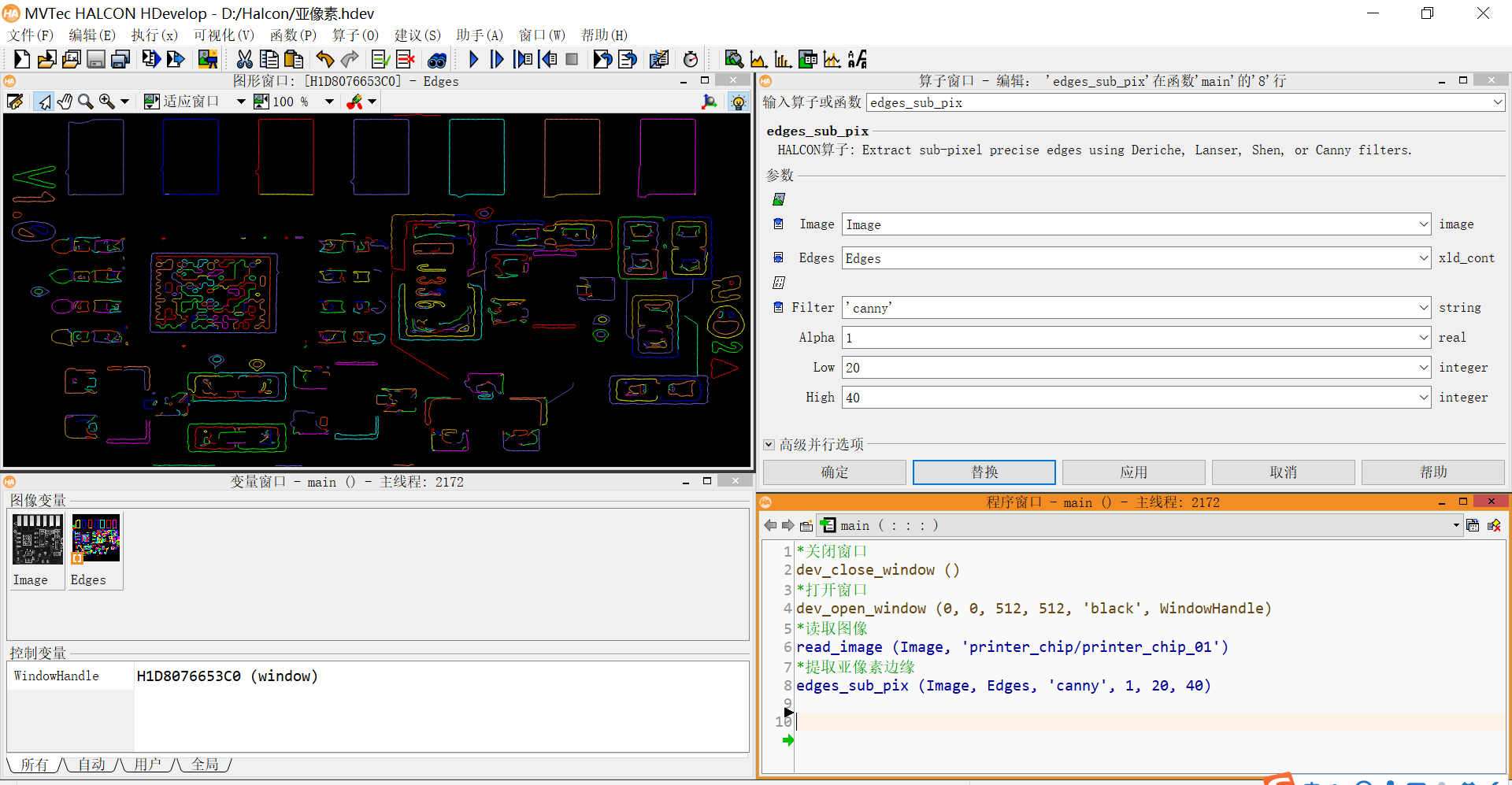• 亚像素边缘检测提取算法的实现
千次阅读
2021-11-29 00:04:27
       亚像素边缘检测提取算法的实现
其中包括插值法、拟合法、基于灰度矩法、基于zernike矩法等


通过Matlab软件运用zernike矩的检测算法
通过如下代码可实现
function zernike7(I)

% 7*7Zernike模板
M00=…
[
0 0.0287 0.0686 0.0807 0.0686 0.0287 0
0.0287 0.0815 0.0816 0.0816 0.0816 0.0815 0.0287
0.0686 0.0816 0.0816 0.0816 0.0816 0.0816 0.0686
0.0807 0.0816 0.0816 0.0816 0.0816 0.0816 0.0807
0.0686 0.0816 0.0816 0.0816 0.0816 0.0816 0.0686
0.0287 0.0815 0.0816 0.0816 0.0816 0.0815 0.0287
0 0.0287 0.0686 0.0807 0.0686 0.0287 0
];
M11R=…
[
0 -0.015 -0.019 0 0.019 0.015 0
-0.0224 -0.0466 -0.0233 0 0.0233 0.0466 0.0224
-0.0573 -0.0466 -0.0233 0 0.0233 0.0466 0.0573
-0.069 -0.0466 -0.0233 0 0.0233 0.0466 0.069
-0.0573 -0.0466 -0.0233 0 0.0233 0.0466 0.0573
-0.0224 -0.0466 -0.0233 0 0.0233 0.0466 0.0224
0 -0.015 -0.019 0 0.019 0.015 0
];
M11I=…
[
0 -0.0224 -0.0573 -0.069 -0.0573 -0.0224 0
-0.015 -0.0466 -0.0466 -0.0466 -0.0466 -0.0466 -0.015
-0.019 -0.0233 -0.0233 -0.0233 -0.0233 -0.0233 -0.019
0 0 0 0 0 0 0
0.019 0.0233 0.0233 0.0233 0.0233 0.0233 0.019
0.015 0.0466 0.0466 0.0466 0.0466 0.0466 0.015
0 0.0224 0.0573 0.069 0.0573 0.0224 0
];
M20=…
[
0 0.0225 0.0394 0.0396 0.0394 0.0225 0
0.0225 0.0271 -0.0128 -0.0261 -0.0128 0.0271 0.0225
0.0394 -0.0128 -0.0528 -0.0661 -0.0528 -0.0128 0.0394
0.0396 -0.0261 -0.0661 -0.0794 -0.0661 -0.0261 0.0396
0.0394 -0.0128 -0.0528 -0.0661 -0.0528 -0.0128 0.0394
0.0225 0.0271 -0.0128 -0.0261 -0.0128 0.0271 0.0225
0 0.0225 0.0394 0.0396 0.0394 0.0225 0
];

if length(size(I))==3 I=rgb2gray(I); end
I=im2bw(I,0.6);
K=double(I);
[m n]=size(K);
xs=double(zeros(m,n));
ys=double(zeros(m,n));

% 卷积运算
A11I=conv2(M11I,K);
A11R=conv2(M11R,K);
A20=conv2(M20,K);

% 截掉多余部分
A11I=A11I(4:end-3,4:end-3);
A11R=A11R(4:end-3,4:end-3);
A20=A20(4:end-3,4:end-3);

J=zeros(size(K));
boundary=J;
theta=atan2(A11I,A11R);%计算theta

%计算边缘的三个参数
A11C=A11R.*cos(theta)+A11I.sin(theta);
l=A20./A11C;
k=1.5
A11C./((1-l.2).1.5);
e=abs(l)>1/3.5;
k(e)=0;

%边缘判断条件
a=abs(l)<1/sqrt(2)*2/7;
b=abs(k)>max(I(😃)/10;
% a,b分别为距离和边缘强度判断结果
J(a&b)=1;
%将图像的最边缘去除
% boundary(2:end-1,2:end-1)=1;
% J(~boundary)=0;
format short
% [x,y]=find(J1);%边缘的像素级坐标
% O=[x y];
% Z=[x+l(find(J
1)).*cos(theta(find(J1))) y+l(find(J1)).*sin(theta(find(J==1)))];%亚像素坐标
% % fprintf(’%.4f %.4f\n’,Z’);

[L,num]=bwlabel(J,8);%对二值图像进行标记
%自动化搜索连通域
s=zeros(1,num);
for i=1:num
s(i)=size(find(L==i),1);
end
[bwL,label]=sort(s,‘descend’);
if label(1)<label(2)
index1=label(1);
index2=label(2);
else
index1=label(2);
index2=label(1);
end

%计算左边探针的最前端坐标
[r1,c1]=find(L==index1);
A1=[r1 c1];
y1=max(A1(:,2));%该连通域中y最大值为针尖处
x1=max(A1(find(A1(:,2)==y1),1));
x1sub=x1+3.5*l(x1,y1)cos(theta(x1,y1));
y1sub=y1+3.5
l(x1,y1)*sin(theta(x1,y1));

%计算最右边探针的最前端坐标
[r2,c2]=find(L==index2);
A2=[r2 c2];
y2=min(A2(:,2));%该连通域中y最小为连通域
x2=max(A2(find(A2(:,2)==y2),1));
x2sub=x2+3.5*l(x2,y2)cos(theta(x2,y2));
y2sub=y2+3.5
l(x2,y2)*sin(theta(x2,y2));

% [x1sub y1sub],[x2sub,y2sub]
subplot(221)
imshow(J)
% figure;
% imcontour(J,1)

% 边界提取
% subplot(122);
% bwimg = bwmorph(J,‘remove’);
% imshow(bwimg)

subplot(222);
I41=imfill(J,‘holes’);
imshow(I41)
title(‘孔洞填充图像’);
% 提取最外围边缘
subplot(223);
I4=bwperim(I41);
imshow(I4); title(‘边缘图像’);
% 去除面积小于150px物体
subplot(224);
I5=bwareaopen(I4,100);
imshow(I5);

更多相关内容
• 这是MATLAB的亚像素边缘提取程序，经过我的验证，能够实现相应的功能。
• 一种快速亚像素边缘检测算法的实现的理论，可以快速实现像素的算法，在时间上有一定的优势，先看理论，然后自己通过理论用C++实现
•图像处理
• 利用正交矩来进行亚像素边缘检测的算法,首先通过计算图像3个不同阶次的Zernike正交矩，把理想阶跃灰度模型的4个参数映射到3个Zernike正交矩中；然后计算边缘所在直线的参数，确定边缘的像素级坐标。
• """ 亚像素处理 """ root2 = np.sqrt(2.0) tri_list = [[0,0,0,0,0,0,0,0],[0,0,0,0,0,0,0,0]] for i in range(kernels_Num): tri_list[i] = np.cos(angle_list[i] * np.pi / 180.0) # sin前面的负号非常关键, ...

本文代码主要是将另外一个博主的c++代码转换成python代码
原文链接：https://blog.csdn.net/yx123919804/article/details/103123071

代码如下：

import time

import cv2
import numpy as np
import datetime

start = time.process_time()

kernels_Num = 8
kernels = ['_' for i in range(kernels_Num)]
kernels = np.array([[1, 2, 1], [0, 0, 0], [-1, -2, -1]], dtype=int)
kernels = np.array([[2, 1, 0], [1, 0, -1], [0, -1, -2]], dtype=int)
kernels = np.array([[1, 0, -1], [2, 0, -2], [1, 0, -1]], dtype=int)
kernels = np.array([[0, -1, -2], [1, 0, -1], [2, 1, 0]], dtype=int)
kernels = np.array([[-1, -2, -1], [0, 0, 0], [1, 2, 1]], dtype=int)
kernels = np.array([[-2, -1, 0], [-1, 0, 1], [0, 1, 2]], dtype=int)
kernels = np.array([[-1, 0, 1], [-2, 0, 2], [-1, 0, 1]], dtype=int)
kernels = np.array([[0, 1, 2], [-1, 0, 1], [-2, -1, 0]], dtype=int)

img_RGB = cv2.cvtColor(img, cv2.COLOR_BGR2RGB)  # 转成RGB 方便后面显示
# 灰度化处理图像
grayImage = cv2.cvtColor(img, cv2.COLOR_BGR2GRAY)

gradients = ['_' for i in range(kernels_Num)]
for i in range(kernels_Num):
"""
显示梯度图像  代码
"""
#     cv2.imshow(str(i),abs)
# cv2.imshow('222',img)
# cv2.waitKey(0)

angle_list = [270, 315, 0, 45, 90, 135, 180, 225]
amplitude = np.full(grayImage.shape,0)
angle = np.full(grayImage.shape,-64)

for r in range(grayImage.shape):
pAmp = amplitude[r]
pAng = angle[r]

pGrad = ['_' for i in range(kernels_Num)]
for i in range(kernels_Num):
for c in range(grayImage.shape):
for i in range(kernels_Num):
pAng[c] = angle_list[i]

"""
显示幅值图像  代码
"""
# cv2.normalize(amplitude,amplitude,0,255,cv2.NORM_MINMAX)
# abs = cv2.convertScaleAbs(amplitude)
# cv2.imshow('amplitude',abs)
# cv2.imshow('222',img)
# cv2.waitKey(0)

"""
显示角度图像  代码
"""
# cv2.normalize(angle,angle,0,255,cv2.NORM_MINMAX)
# abs = cv2.convertScaleAbs(angle)
# cv2.imshow('angle',abs)
# cv2.imshow('222',img)
# cv2.waitKey(0)

edge = np.full(grayImage.shape,0)
edge.astype('uint8')
thres = 100 #阈值  设置最小幅度值
for r in range(1, grayImage.shape-1):
pAmp1 = amplitude[r-1]
pAmp2 = amplitude[r]
pAmp3 = amplitude[r+1]

pAng = angle[r]
pEdge = edge[r]
for c in range(1, grayImage.shape-1):

if (pAmp2[c] < thres):
continue
if pAng[c] == 270:
if pAmp2[c] > pAmp1[c] and pAmp2[c] >= pAmp3[c]:
pEdge[c] = 255
elif pAng[c] == 90:
if pAmp2[c] >= pAmp1[c] and pAmp2[c] > pAmp3[c]:
pEdge[c] = 255
elif pAng[c] == 315:
if pAmp2[c] > pAmp1[c - 1] and pAmp2[c] >= pAmp3[c + 1]:
pEdge[c] = 255
elif pAng[c] == 135:
if pAmp2[c] >= pAmp1[c - 1] and pAmp2[c] > pAmp3[c + 1]:
pEdge[c] = 255
elif pAng[c] == 0:
if pAmp2[c] > pAmp2[c - 1] and pAmp2[c] >= pAmp2[c + 1]:
pEdge[c] = 255
elif pAng[c] == 180:
if pAmp2[c] >= pAmp2[c - 1] and pAmp2[c] > pAmp2[c + 1]:
pEdge[c] = 255
elif pAng[c] == 45:
if pAmp2[c] >= pAmp1[c + 1] and pAmp2[c] > pAmp3[c - 1]:
pEdge[c] = 255
elif pAng[c] == 225:
if pAmp2[c] > pAmp1[c + 1] and pAmp2[c] >= pAmp3[c - 1]:
pEdge[c] = 255
"""
显示单像素图像
"""
# edge = cv2.convertScaleAbs(edge)
# cv2.imshow('edge',edge)
# cv2.imwrite('edge.png', edge)
# cv2.waitKey(0)

"""
亚像素处理
"""
root2 = np.sqrt(2.0)
tri_list = [[0,0,0,0,0,0,0,0],[0,0,0,0,0,0,0,0]]
for i in range(kernels_Num):
tri_list[i] = np.cos(angle_list[i] * np.pi / 180.0)
# sin前面的负号非常关键, 因为图像的y方向和直角坐标系的y方向相反
tri_list[i] = -np.sin(angle_list[i] * np.pi / 180.0)
vPts = []
for r in range(1, grayImage.shape-1):
pAmp1 = amplitude[r - 1]
pAmp2 = amplitude[r]
pAmp3 = amplitude[r + 1]

pAng = angle[r]
pEdge = edge[r]
for c in range(1, grayImage.shape-1):
if (pEdge[c]):
nAngTmp = 0
dTmp = 0
if pAng[c] == 270:
nAngTmp = 0
dTmp = (pAmp1[c] - pAmp3[c]) / (pAmp1[c] + pAmp3[c] - 2 * pAmp2[c]) * 0.5
# print([c + dTmp * tri_list[nAngTmp],r + dTmp * tri_list[nAngTmp]])
elif pAng[c] == 90:
nAngTmp = 4
dTmp = -(pAmp1[c] - pAmp3[c]) / (pAmp1[c] + pAmp3[c] - 2 * pAmp2[c]) * 0.5
elif pAng[c] == 315:
nAngTmp = 1
dTmp = (pAmp1[c - 1] - pAmp3[c + 1]) / (pAmp1[c - 1] + pAmp3[c + 1] - 2 * pAmp2[c]) * root2 * 0.5
elif pAng[c] == 135:
nAngTmp = 5
dTmp = -(pAmp1[c - 1] - pAmp3[c + 1]) / (pAmp1[c - 1] + pAmp3[c + 1] - 2 * pAmp2[c]) * root2 * 0.5
elif pAng[c] == 0:
nAngTmp = 2
dTmp = (pAmp2[c - 1] - pAmp2[c + 1]) / (pAmp2[c - 1] + pAmp2[c + 1] - 2 * pAmp2[c]) * 0.5
elif pAng[c] == 180:
nAngTmp = 6
dTmp = -(pAmp2[c - 1] - pAmp2[c + 1]) / (pAmp2[c - 1] + pAmp2[c + 1] - 2 * pAmp2[c]) * 0.5
elif pAng[c] == 45:
nAngTmp = 3
dTmp = (pAmp3[c - 1] - pAmp1[c + 1]) / (pAmp1[c + 1] + pAmp3[c - 1] - 2 * pAmp2[c]) * root2 * 0.5
elif pAng[c] == 225:
nAngTmp = 7
dTmp = -(pAmp3[c - 1] - pAmp1[c + 1]) / (pAmp1[c + 1] + pAmp3[c - 1] - 2 * pAmp2[c]) * root2 * 0.5

x = c + dTmp * tri_list[nAngTmp]
y = r + dTmp * tri_list[nAngTmp]
vPts.append([x,y])
"""
输出亚像素坐标
"""
# for x,y in vPts:
#     print(x,y)

endtime = time.process_time()
print (str(endtime - start) + 's')


加了执行时间

展开全文opencv 计算机视觉 python
• 利用opencv的亚像素级别的边缘检测和获取，添加了原有程序的包含文件和库，可以直接调试运行，个边参数可以根据实际情形修改
• 亚像素边缘提取原理：采用插值算法，精确定位边缘的位置。 Halcon提供了亚像素边缘提取方法，可直接获取高精度的像素边缘。 算子：edges_sub_pix () 像素边缘 *关闭窗口 dev_close_window () *打开...

目录

• 概念
• 算子
• 代码
• 实例

1.概念

亚像素：原先的像素单位为1，变为比1小的精度。亚像素比像素精度更高。

亚像素边缘提取原理：采用插值算法，精确定位边缘的位置。2.算子

Halcon提供了亚像素边缘提取方法，可直接获取高精度的亚像素边缘。

算子：edges_sub_pix ()   亚像素边缘

3.代码

*关闭窗口
dev_close_window ()

*打开窗口
dev_open_window (0, 0, 512, 512, 'black', WindowHandle)

*读取图像

*提取亚像素边缘
edges_sub_pix (Image, Edges, 'canny', 1, 20, 40)



4.实例展开全文Halcon
• ## 亚像素边缘提取方法总结

万次阅读 多人点赞 2017-04-11 10:08:37
亚像素边缘技术概述 数字图像的边缘检测是图像分割、目标识别、区域形状提取等图像处理领域的重要基础。在进行图像理解和分析时，第一步往往是边缘检测。 1965年，L．G．Roberts最早开始系统研究边缘检测。最早提出...

# 亚像素边缘技术概述

数字图像的边缘检测是图像分割、目标识别、区域形状提取等图像处理领域的重要基础。在进行图像理解和分析时，第一步往往是边缘检测。从广义来讲，边缘形成的是对象的轮廓，对象是视觉系统的分析主体。

1965年，L．G．Roberts最早开始系统研究边缘检测。最早提出的是一阶微分算子，1965年L．G．Roberts提出Robert算子，随后，在Robert算子基础上人们经过改进得到的Sobel算子、Prcwitt 算子和Kirsh 算子等。但是，这些算子检测到的边缘往往不是很理想，边缘较宽，还需要进行细化处理，这样又影响到边缘的定位。在这种情况下，Laplacian 算子应运而生。这种算子利用二阶导数的过零点来检测边缘位置，所得边缘较细，不需要边缘细化，定位精确度也相应的得到了提高。我们在研究图像的边缘时，不可避免的会遇到噪声的干扰。用微分算子法可以检测图像的边缘同时也会检测到噪声，为了减少噪声的干扰，人们很自然地提出在进行边缘检测之前对图像进行适当的平滑滤波。基于这种思想Mart和Hildreth提出了LOG(Laplacian of Gaussian)算子。随着研究的进一步深入，后来Canny J F指出高斯函数的一阶导数可以近似为最优边缘检测算子，基于这种思想，提出Canny算子，这种算子具有较理想的检测标准、定位标准和单响应标准。

上述传统的边缘检测方法的检测精度最高只能达到一个像素级，但是，随着科学技术的飞速发展，工业检测等应用对精确度的要求不断提高，传统的像素级边缘检测方法已经不能满足实际测量的需要。在此基础上，亚像素级边缘提取技术应运而生。

亚像素是将像素这个基本单位再进行细分，它是比像素还小的单位，从而提高了图像分辨率。通常情况下，亚像素边缘点存在于图像中逐渐发生过度变化的区域，我们可以利用多项式拟合等多种方法获得边缘点的亚像素位置。亚像素定位可以理解为在摄像系统硬件条件不变的情况下，用软件算法来提高边缘检测精度的方法，或者说是一种使分辨率小于一个像素的图像处理技术。

亚像素定位技术的应用具有一定的前提条件

1、被检测目标不是由孤立的、单个的像素点组成，而是由多个像素点组成，且这些像素点应具有一定的分布特性，如灰度分布、几何形状分布特性等；

2、一般情况下，不同的目标都具有各自的特征，主要包括基于目标的灰度分布特征、几何形状特征、几何与灰度耦合特征等，能够分析并利用已知的目标特征，通过对被检测目标图像的分析、识别，最后确定出目标的准确位置。在此分析定位的过程中，采用浮点运算对目标图像进行定位，得到的目标定位精度高于整像素级的定位精度。这种利用目标特性从图像中分析，计算出最符合此特征的目标位置的方法称为图像目标亚像素定位技术。

# 亚像素边缘技术国内外发展现状

目前研究的亚像素级的边缘检测算法，可以归纳为3种类型:矩方法、插值法和拟合法。

## 矩方法

矩作为数学上的完备描述,相当于原函数在新的坐标空间上的展开,即一个分段连续有界函数可用其矩族唯一表示。灰度矩边缘定位算法的基本原理是假设实际图像中的实际边缘分布与理想边缘模型的灰度矩保持一致，即矩不变。通过此关系来确定实际边缘的位置。Tabatabai等首先提出一种利用前三阶灰度矩对边缘进行亚像素边缘定位的算法 ,随后基于空间矩、Zernike正交矩的方法也相继被提出。Zernike矩的方法由于只需要计算3个模板，计算量比空间矩的方法要小得多。但是 ,这些方法都是针对理想边缘模型提出的。Shan等对矩方法进行了改进，使用了模糊边缘模型，更能真实反映边缘信息。矩方法的优点是计算简便，并且可以得到解析解。但是矩方法对图像噪声敏感，如果考虑模糊后的边缘模型，就会增加模型参数，使得解析解的确定变得十分困难。

下面文章是介绍zernike矩方法边缘提取的：

http://www.cnblogs.com/luo-peng/p/5171455.html

http://blog.csdn.net/u010839382/article/details/50610459

## 插值法

插值法的核心是对像素点的灰度值或灰度值的导数进行插值，增加信息，以实现亚像素边缘检测。其中，研究比较多的方法有二次插值、B样条插值和切比雪夫多项式插值等。插值类的运算时间短，二次插值算法简单，可以通过硬件实现，适合在线检测。当光学系统的线扩散函数对称时，插值边缘检测的精度较高。插值法的特点同基于矩的方法类似，计算过程简单，但是容易受噪声的影响。

## 拟合法

拟合方法是通过对假设边缘模型灰度值进行拟合来获得亚像素的边缘定位。Nalwa等给出一种边缘模型为双曲正切函数的最小二乘拟合算法；Ye等提出的算法所用的边缘模型是理想边缘模型与高斯函数卷积得到的高斯型边缘函数。这两种算法都能提供较高的亚像素边缘定位精度。由于拟合不需要数值微分，而且按各灰度值到拟合曲线的距离最小进行拟合，不但合理地利用了有误差的灰度值，又可以减小灰度值误差的影响，因此拟合方法对噪声不敏感。但因模型复杂，其求解速度慢。

## 基于改进形态学梯度和Zernike矩的亚像素边缘检测方法

该算法先利用改进的数学形态学梯度算子进行边缘点的粗定位，在像素级上确定边缘点的坐标和梯度方向；然后再根据构造的边缘点向量和参考阈值，用Zernike矩算法对边缘点进行亚像素的重新定位，实现图像的亚像素边缘检测。这种复合的图像边缘亚像素检测算法，能够很好地融合数学形态学梯度算子及Zernike矩算法的优点，具备良好的抗噪性能与亚像素精确定位能力，其计算量相对较少，能快速实现CCD图像测量系统的亚像素边缘检测。此外，由于一般测量图像简单且对比度高，若采用适当的模板窗口，该算法可具有良好的处理效率，能够满足一般图像测量系统的实时性、亚像素精确测量要求，具备良好的应用前景。

## 基于改进的形态学梯度的样条插值亚像素边缘检测方法

此改进方法是将改进的形态学梯度滤波算子与三次样条插值法结合起来进行边缘检测，首先利用改进的数学形态学梯度滤波算子进行边缘点的粗定位，得到图像的像素级边缘；然后再利用三次样条插值法对提取出的边缘图像进行插值运算，即亚像素精定位。最后将插值后的边缘进行细化，可得到亚像素级边缘图像。

比较经典的亚像素提取论文subpixel-precise extranction of lines and edges》，

参考：

http://blog.csdn.net/lsh_2013/article/details/44980993

http://blog.csdn.net/kezunhai/article/details/52248015 （亚像素级提取的例子）

http://blog.csdn.net/augusdi/article/details/12907151/

展开全文• 在分析Tabatabai提出的灰度矩亚像素边缘检测算法的基础上，指出灰度矩算法存在 边缘判断条件不够完善和未能考虑模板效应的问题，提出了改进方法，考虑Tabatabai的灰度矩算 法产生很多虚假边缘，改进算法分析了各参数...图像处理
• 1 引言  数字图像的边缘检测是图像分割、目标...传统的边缘检测方法的检测精度最高只能达到一个像素级，但是，随着科学技术的飞速发展，工业检测等应用对精确度的要求不断提高，传统的像素边缘检测方法已经不能满
• 但是,我怀疑你能用这些图像实现1/100像素亚像素精度,原因如下： >正如您在配置文件中看到的那样,您的白人已经饱和(灰度级为255).当你削减真正的atan轮廓时,合身是有偏见的.如果您可以控制实验,我建议您再次使用较...
• 基于多项式插值的亚像素边缘定位算法 OpenCV, C++, 有图有真相OpenCV C++
• 首先使用canny或其他方法将图片边缘轮廓提取： （下图为mask处理后的图像） ...之后根据八邻域算法进行改进，提出了一种边缘亚像素点连线的方法： import random import cv2 from tqdm import tqdmc语言 数据结构 python
• 比如说你在亚太赛得A题这个图： 解决方案还可以采用这个热大佬得方法，并不是我写的，学一下哇。 首先，配置opencv,我写了教程： ...基于多项式的亚像素边缘定位算法 我还没测试）有空再给你们测试吧，自己试试。 ...matlab 开发语言
• 一种改进的正交 Fourier-Mellin 矩亚像素边缘检测算法 [C......本文提出一种新的像素精度的边缘检测算法 . 该方法给出了一种可修正的贝塞尔点扩...一种亚像素边缘检测方法_IT/计算机_专业资料。提出一种新的亚像素...
• 快速亚像素边缘提取算法   关键词： Facet模型，曲面拟合，离散切比雪夫正交多项式，像素边缘             特色书店，图像处理，机器视觉，C/C++，控制理论/工程，工程数学..... ...图像处理
• 首先，建立亚像素边缘模型，利用各级 Franklin 矩的卷积来提取图像边缘点的细节特征;然后，依据Franklin 矩的旋转不变性原理，分析图像边缘旋转至垂直方向后各级 Franklin 矩之间的关系，从而确定图像中亚像素边缘的...
• ## 亚像素级点定位及边缘定位算法

万次阅读 多人点赞 2017-02-03 21:15:22
在这篇博客里，主要讲解两点：点定位(Point localization)以及边缘定位(Edge localization)，均是亚像素级定位精度。当然还有其他定位方法，这里仅针对亮点和边缘两种情形分别进行精确定位。 1. 为何需要进行...
• 精确确定数字图像边缘的位置,对于图像测量非常重要。同时,图像获取过程中受到各种噪声的影响, 必然会引起图像边缘的模糊,因此对图像边缘提取技术的研究一直...介绍了基于曲面拟合的和基于灰度矩的亚像素边缘检测算法
• 一般在做项目时，无论是边缘检测、瑕疵检测、Blob检测等应用，都有一定的通用套路，也可以说是通用处理框架，在此框架下根据具体应用具体分析，基本上所有项目都可完成。 总结起来：通用套路 + 灵活运用 对于...halcon教程
• Devernay像素校正算法 Canny检测出的边缘是像素级别的，而有时需要精度低于一个像素，即亚像素边缘提取，Devernay【3】在Canny算法的基础上进行了拓展：将新的边缘点定义为相邻几个梯度模值差值的最大值，可以...opencv
• 针对传统边缘检测算法存在定位精度低、对噪声敏感等...形态学细化算子将提取出的边缘进行细化，可有效地检测出图像边缘，实现亚像素边缘 检测。实验结果表明，这种方法能准确地检测出边缘，优于传统的边缘检测方法。
• 本发明专利技术提供一种亚像素边缘检测方法，包括如下步骤：获取图像；图像预处理；像素计算；生成亚像素边缘。本发明专利技术采用了像素的像素处理策略，有效地摆脱了由于像素点过大造成的精度丢失问题，能找到...
• 基于灰度矩的亚像素边缘检测，精确度可以达到0.1-0.2个像素，内附源代码及主要参考文献，仅供学习参考！
• 对于光照不均匀的图像，形态学边缘提取算法的分辨率远远逊色于人眼的分辨率。产生这种差异的原因是形态学算法仅仅是从几何学的角度出发来检测边缘，并没有模拟出人眼的生物特性。为了提高形态学算法的分辨率，通过...
• 第五节：亚像素边缘提取&相关算子 概念：摄像机拍摄时，将物理世界中连续的图像进行了离散化处理。到成像面上每一个像素点只代表其附近的颜色。两个像素之间有5.2微米的距离，在宏观上可以看作是连在一起的。...
• 目录1 基于灰度矩的亚像素边缘检测理论2 MATLAB实现2.1 main.m2.2 Conv.m2.3 Zhongxin.m 【代码下载】 1 基于灰度矩的亚像素边缘检测理论 参考文献：亚像素边缘检测技术研究_张美静 2 MATLAB实现 2.1 main.m ......